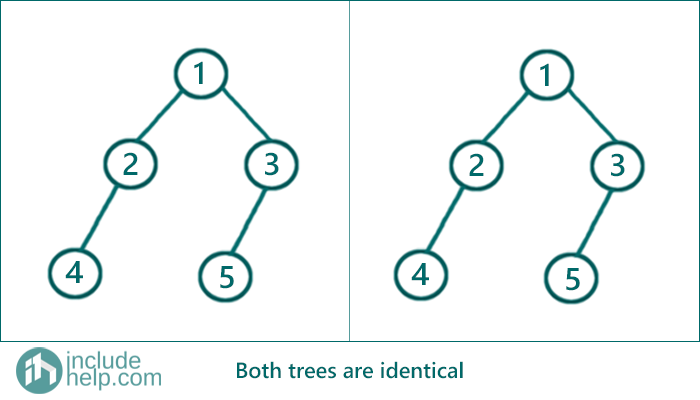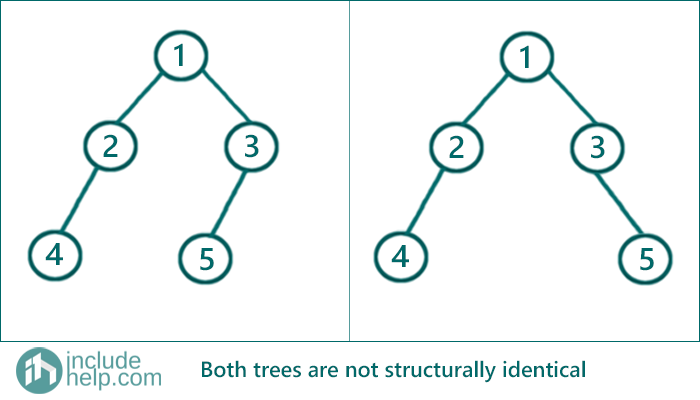# Check if two trees are identical or not

In this article, we are going to see how to check two trees are identical or not?
Submitted by Radib Kar, on August 07, 2020

In this article, we going to see given two Tree roots how we can find whether two trees are identical or not.

## When we can say two trees are identical?

For two trees are said to be identical the roots have to be same (identical value) and both their subtrees should be equal.

Say if identical is a function that takes two tree nodes and returns true or false based on where they are identical or not, then based on the above inference we can write like below

identical(root1, root2) returns ((root1->val==root2->val) && identical(root1->left,root2->left) && identical(root1->right,root2->right))

That means it returns true only if root values are same and both their left subtrees are same and both their right subtrees are the same. Otherwise, it returns false.

To check whether both their left and right subtrees are equal or not we used the same function recursively.

Recursive function to check

```bool identical(TreeNode* root1,TreeNode* root2){
/////base cases/////
//1.both roots are NULL then they are identical
if (both roots are NULL)
return true;
//2.one root is NULL & other is not, then they are not identical
if (one root is NULL and another is not)
return false;

//if root values are different they can't be identical
if(root values are different)
return false;

//if root values are same then recursively check their subtrees.
//If both subtrees are identical then it will return true only
return identical(root1->left,root2->left) && identical(root1->right,root2->right);
}
```

Example

1) When two trees are identical

Below is the example where both the trees are identical.If we run a dry-run on the above tree. Then it starts with calling identical (1,1)

```identical(1,1)
Since no root is NULL, it doesn’t enter the base cases
Both root has same value that is 1
So, it now checks for both subtree,
call to identical(1->left, 1->left) && identical(1->right, 1->right)

identical(1->left, 1->left):
identical(2,2)
Since no root is NULL, it doesn’t enter the base cases
Both root has same value that is 2
So, it now checks for both subtree,
call to identical(2->left, 2->left) && identical(2->right, 2->right)

identical(2->left, 2->left):
identical(4,4)
Since no root is NULL, it doesn’t enter the base cases
Both root has same value that is 4
So, it now checks for both subtree,
call to identical(4->left, 4->left) && identical(4->right, 4->right)
Since, identical(4->left, 4->left) returns true
as both are NULL(4->left).
Same way, identical(4->right, 4->right) returns true

So, the entire function identical(2->left,2->left) returns true,
that means the left subtree of root1(2) & root2(2) are equal
Similarly,
We can execute identical(2->right, 2->right) and
that will return true also.
So now identical(1->left, 1->left) returns true
that means left subtree of our original roots are equal.

We can find right subtrees are also identical,
Hence both trees are identical.
```

2) When the two trees are not identical

Below is the example where the trees are not identical.If we run a dry-run on the above tree. Then it starts with calling identical (1,1)

You can run a dry run of course, but since the length of the article will increase, I am skipping. But to point out why the above trees are not identical and how our algorithm with detect

We can say that at the stage of calling identical(3,3) it will return false since the left child of 3 is 5 for the first tree whereas it's NULL for the second tree. So it will hit the second base case and return false. Once a function call returns false that will bubble up to the first function call and ultimately lead to an output false.

C Implementation:

```#include <stdio.h>
#include <stdlib.h>

struct tree {
int val;
struct tree* left;
struct tree* right;
};
typedef struct tree TreeNode;

TreeNode* newTree(int data)
{
// Allocate memory for new node
TreeNode* root = (TreeNode*)malloc(sizeof(TreeNode));

// Assign data to this node val
root->val = data;

// Initialize left and right children as NULL
root->left = NULL;
root->right = NULL;
return (root);
}

int identical(TreeNode* root1, TreeNode* root2)
{
/////base cases/////
//1.both roots are NULL then they are identical
if (root1 == NULL && root2 == NULL)
return 1;
//2.one root is NULL & other is not, then they are not identical
if (root1 == NULL || root2 == NULL)
return 0;

//if root values are different they can't be identical
if (root1->val != root2->val)
return 0;

//if root values are same then recursively check their subtrees

return identical(root1->left, root2->left) && identical(root1->right, root2->right);
}

int main()
{
//building the trees like example 1
TreeNode* root1 = newTree(1);
root1->left = newTree(2);
root1->right = newTree(3);
root1->left->left = newTree(4);
root1->right->left = newTree(5);

TreeNode* root2 = newTree(1);
root2->left = newTree(2);
root2->right = newTree(3);
root2->left->left = newTree(4);
root2->right->left = newTree(5);
if (identical(root1, root2))
printf("Both the trees in example 1 are identical\n");
else
printf("Both the trees in example 1 are not identical\n");

//building the trees like example 2
TreeNode* root3 = newTree(1);
root3->left = newTree(2);
root3->right = newTree(3);
root3->left->left = newTree(4);
root3->right->left = newTree(5);

TreeNode* root4 = newTree(1);
root4->left = newTree(2);
root4->right = newTree(3);
root4->left->left = newTree(4);
root4->right->right = newTree(5);

if (identical(root3, root4))
printf("Both the trees in example 2 are identical\n");
else
printf("Both the trees in example 2 are not identical\n");

return 0;
}
```

Output:

```Both the trees in example 1 are identical
Both the trees in example 2 are not identical
```

C++ Implementation:

```#include <bits/stdc++.h>
using namespace std;

// tree node is defined
class TreeNode {
public:
int val;
TreeNode* left;
TreeNode* right;
TreeNode(int data)
{
val = data;
left = NULL;
right = NULL;
}
};

bool identical(TreeNode* root1, TreeNode* root2)
{
/////base cases/////
//1.both roots are NULL then they are identical
if (root1 == NULL && root2 == NULL)
return true;
//2.one root is NULL & other is not, then they are not identical
if (root1 == NULL || root2 == NULL)
return false;

//if root values are different they can't be identical
if (root1->val != root2->val)
return false;

//if root values are same then recursively check their subtrees

return identical(root1->left, root2->left) && identical(root1->right, root2->right);
}

int main()
{
//building the trees like example 1
TreeNode* root1 = new TreeNode(1);

root1->left = new TreeNode(2);
root1->right = new TreeNode(3);
root1->left->left = new TreeNode(4);
root1->right->left = new TreeNode(5);

TreeNode* root2 = new TreeNode(1);
root2->left = new TreeNode(2);
root2->right = new TreeNode(3);
root2->left->left = new TreeNode(4);
root2->right->left = new TreeNode(5);
if (identical(root1, root2))
cout << "Both the trees in example1 are identical\n";
else
cout << "Both the trees in example1 are not identical\n";

//building the trees like example 2
TreeNode* root3 = new TreeNode(1);
root3->left = new TreeNode(2);
root3->right = new TreeNode(3);
root3->left->left = new TreeNode(4);
root3->right->left = new TreeNode(5);

TreeNode* root4 = new TreeNode(1);
root4->left = new TreeNode(2);
root4->right = new TreeNode(3);
root4->left->left = new TreeNode(4);
root4->right->right = new TreeNode(5);
if (identical(root3, root4))
cout << "Both the trees in example 2 are identical\n";
else
cout << "Both the trees in example 2 are not identical\n";

return 0;
}
```

Output:

```Both the trees in example1 are identical
Both the trees in example 2 are not identical
```

What's New (MCQs)

Top Interview Coding Problems/Challenges!

Languages: » C » C++ » C++ STL » Java » Data Structure » C#.Net » Android » Kotlin » SQL
Web Technologies: » PHP » Python » JavaScript » CSS » Ajax » Node.js » Web programming/HTML
Solved programs: » C » C++ » DS » Java » C#
Aptitude que. & ans.: » C » C++ » Java » DBMS
Interview que. & ans.: » C » Embedded C » Java » SEO » HR
CS Subjects: » CS Basics » O.S. » Networks » DBMS » Embedded Systems » Cloud Computing
» Machine learning » CS Organizations » Linux » DOS
More: » Articles » Puzzles » News/Updates

© https://www.includehelp.com some rights reserved.